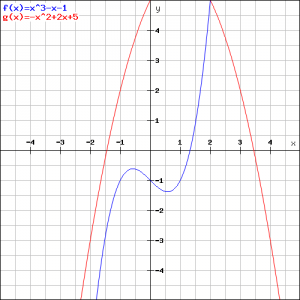# Solution assignment 07 Tangent line to graph

### Assignment 7

Calculate the equation of the tangent line(s) of the graph of the function: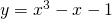parallel to the tangent line at the point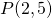of the graph of the function: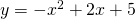### Solution

First we calculate the slope of the tangent line at. For this we have to differentiate the second function: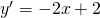In the pointthe slope is equal to:Now we have to try and find the points on the graph of the function:of which the tangent line(s) has (have) a slope equal to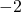.
An tangent line at the graph of this function has a slope: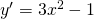So we have to find the values offor which we have: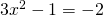or: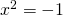This equation has no (real) solutions and thus the required tangent line(s) does (do) not exist. We can verify this in the figure below.